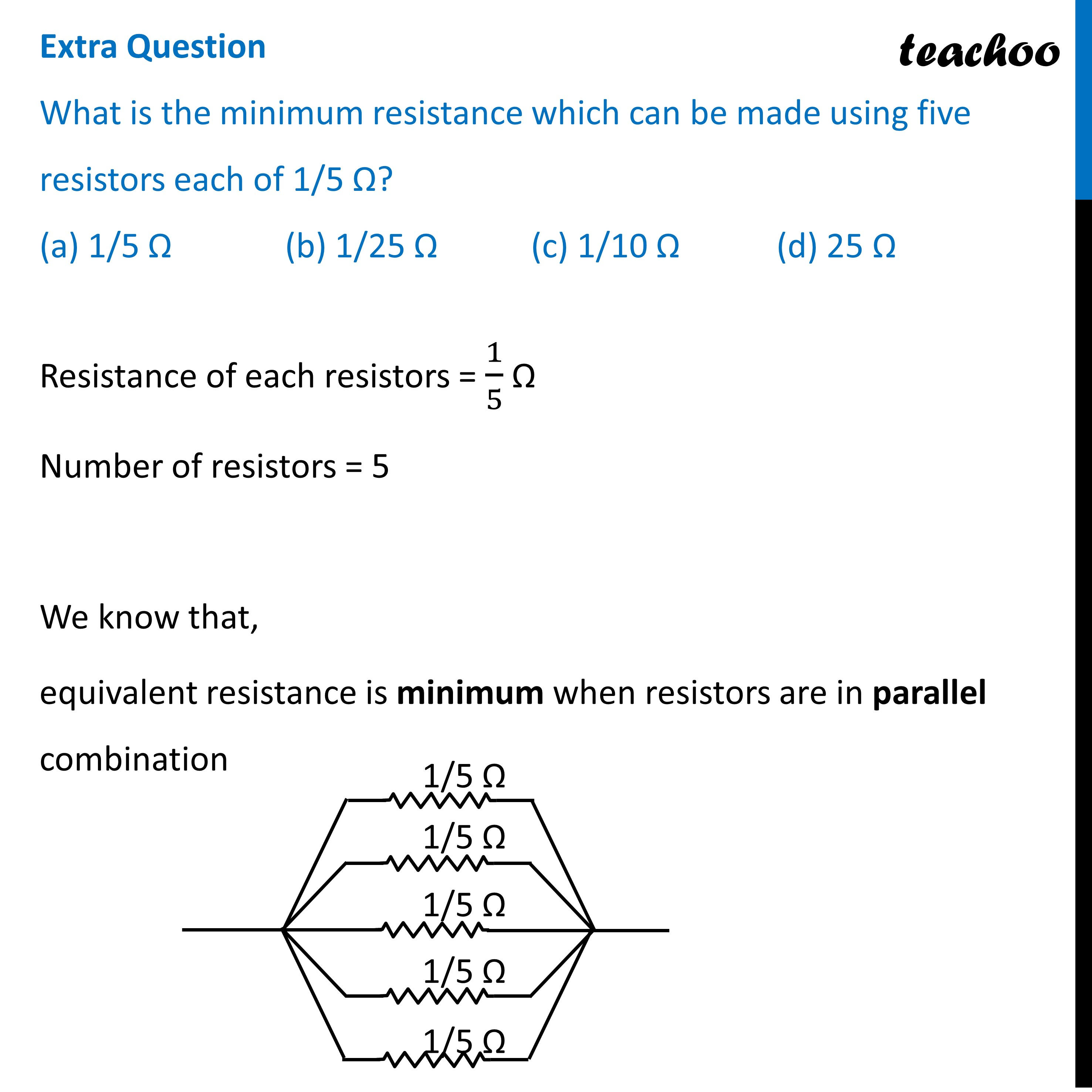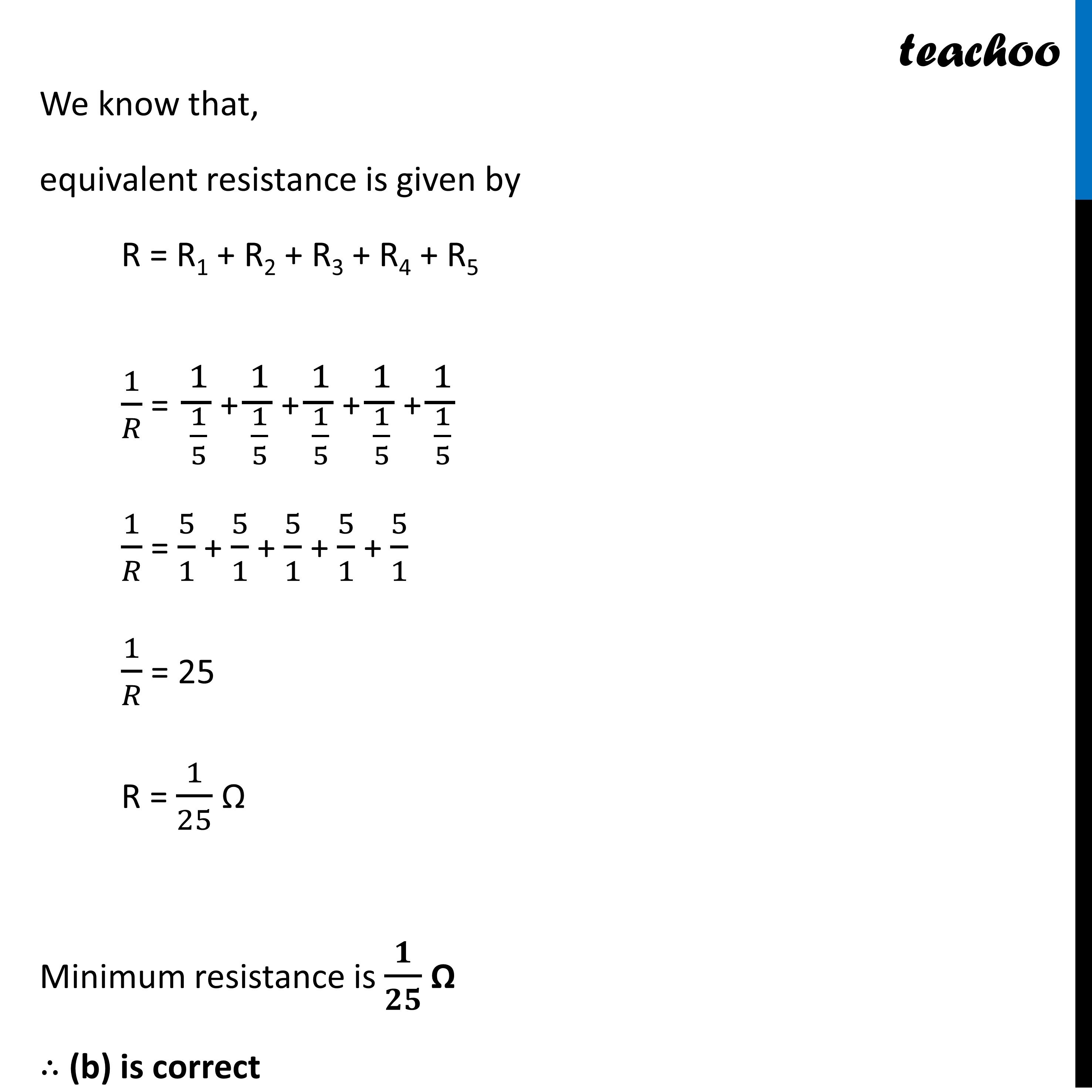MCQs from (Past Year Papers & NCERT Exemplar)

Class 10
Chapter 12 Class 10 - Electricity

## (d) 25 Ω.Learn in your speed, with individual attention - Teachoo Maths 1-on-1 Class

### Transcript

Extra Question What is the minimum resistance which can be made using five resistors each of 1/5 Ω? (a) 1/5 Ω (b) 1/25 Ω (c) 1/10 Ω (d) 25 ΩResistance of each resistors = 1/5 Ω Number of resistors = 5 We know that, equivalent resistance is minimum when resistors are in parallel combination We know that, equivalent resistance is given by R = R1 + R2 + R3 + R4 + R5 1/𝑅 = 1/(1/5) + 1/(1/5) + 1/(1/5) + 1/(1/5) + 1/(1/5) 1/𝑅 = 5/1 + 5/1 + 5/1 + 5/1 + 5/1 1/𝑅 = 25 R = 1/25 Ω Minimum resistance is 𝟏/𝟐𝟓 Ω ∴ (b) is correct